# RS Aggarwal Solutions for Class 10 Maths Chapter 2 Polynomials Exercise 2B

R S Aggarwal Solutions for Class 10 Maths Chapter 2 Polynomials, contains solutions for all exercise 2B questions. Chapter 2 RS Aggarwal solutions on exercise 2b is based on polynomials and relation between the zeros and coefficients of a quadratic Polynomial. Students can download the R S Aggarwal Solutions of Class 10 and start practicing offline.

### Download PDF of RS Aggarwal Solutions for Class 10 Maths Chapter 2 Polynomials Exercise 2B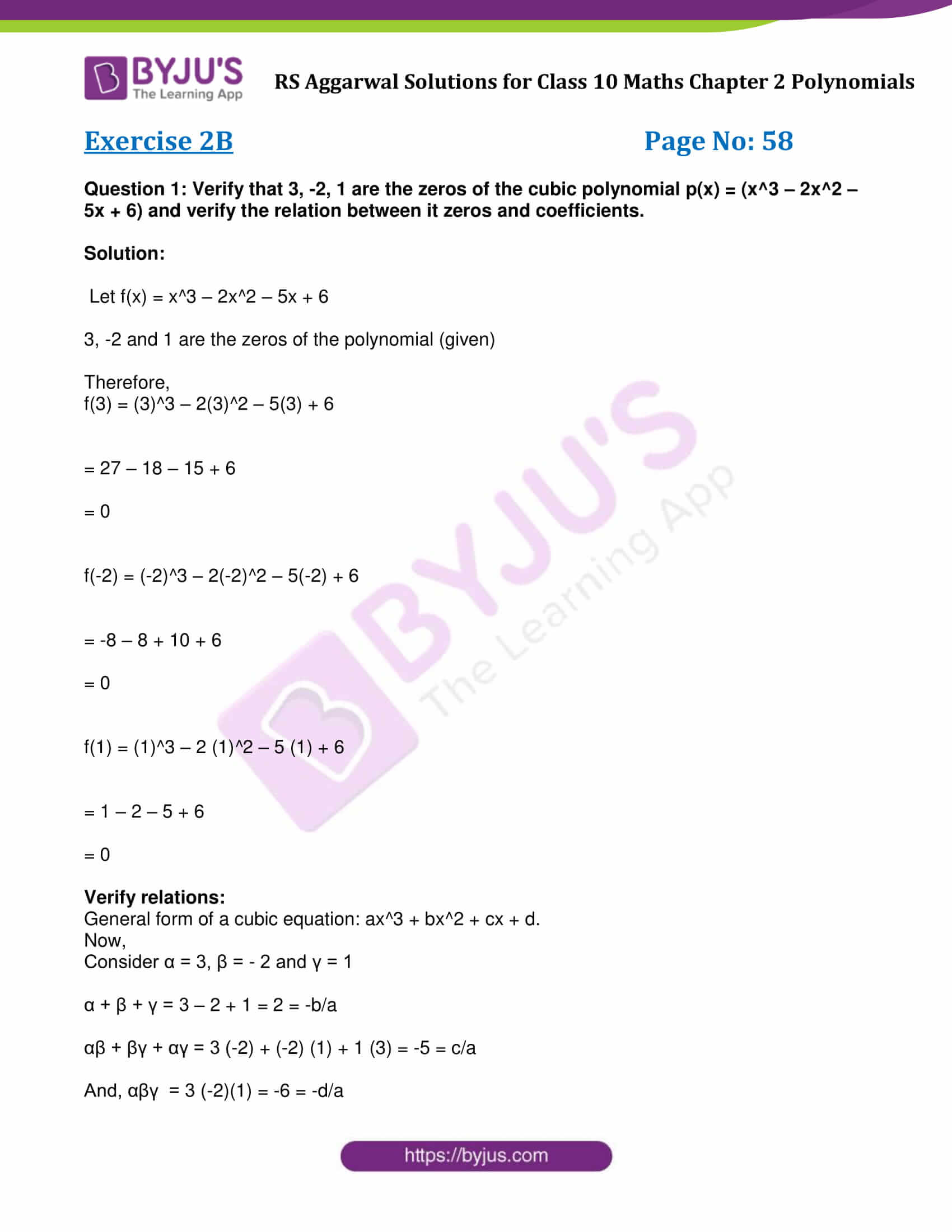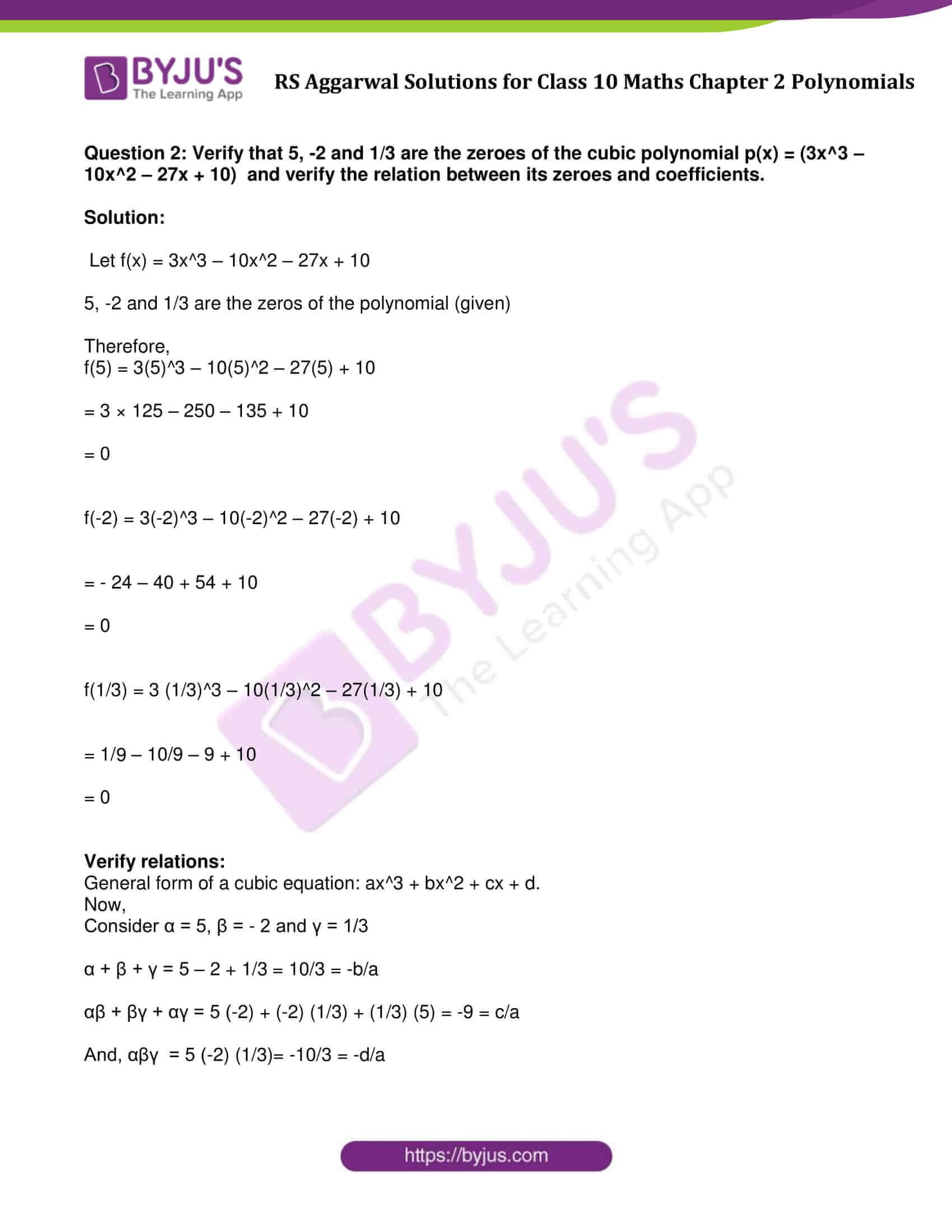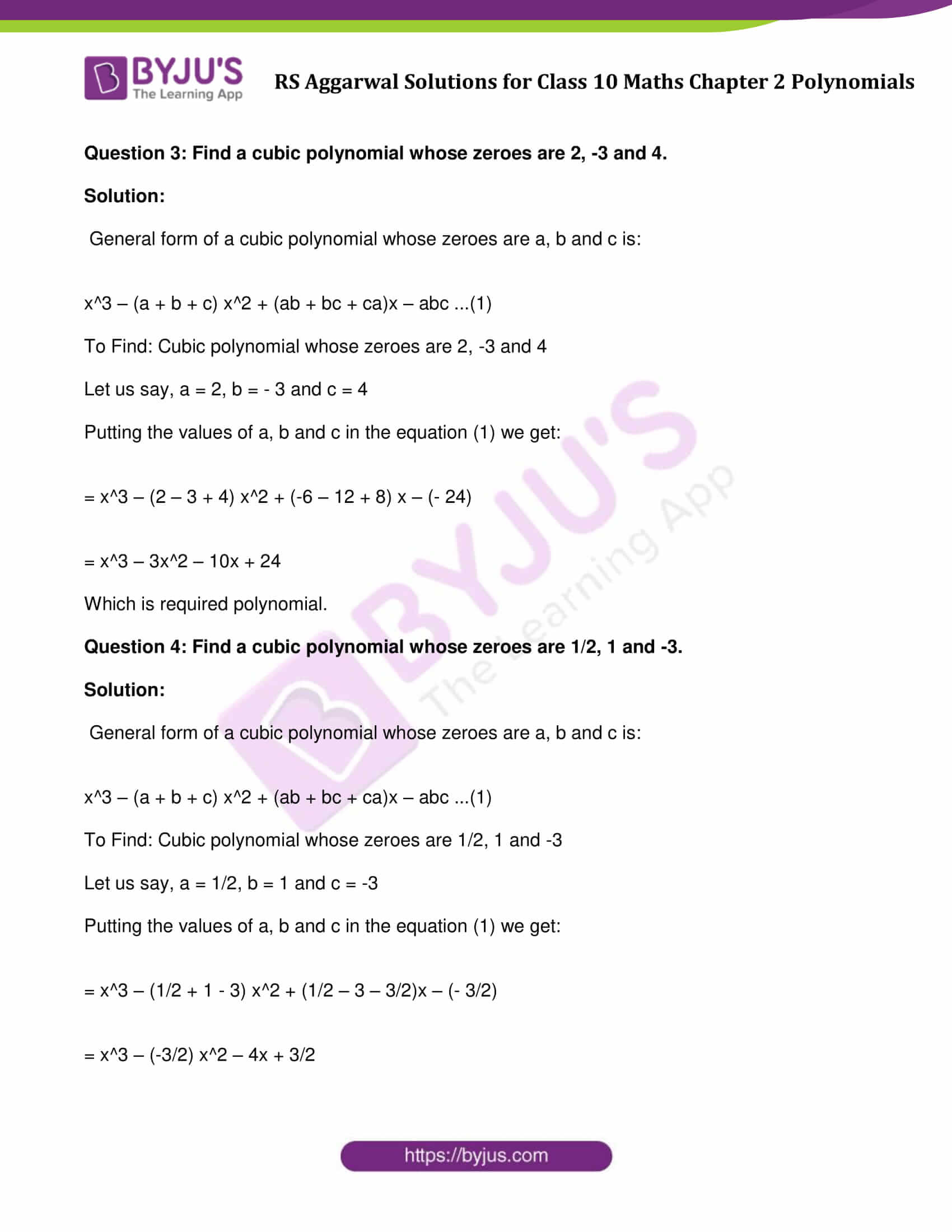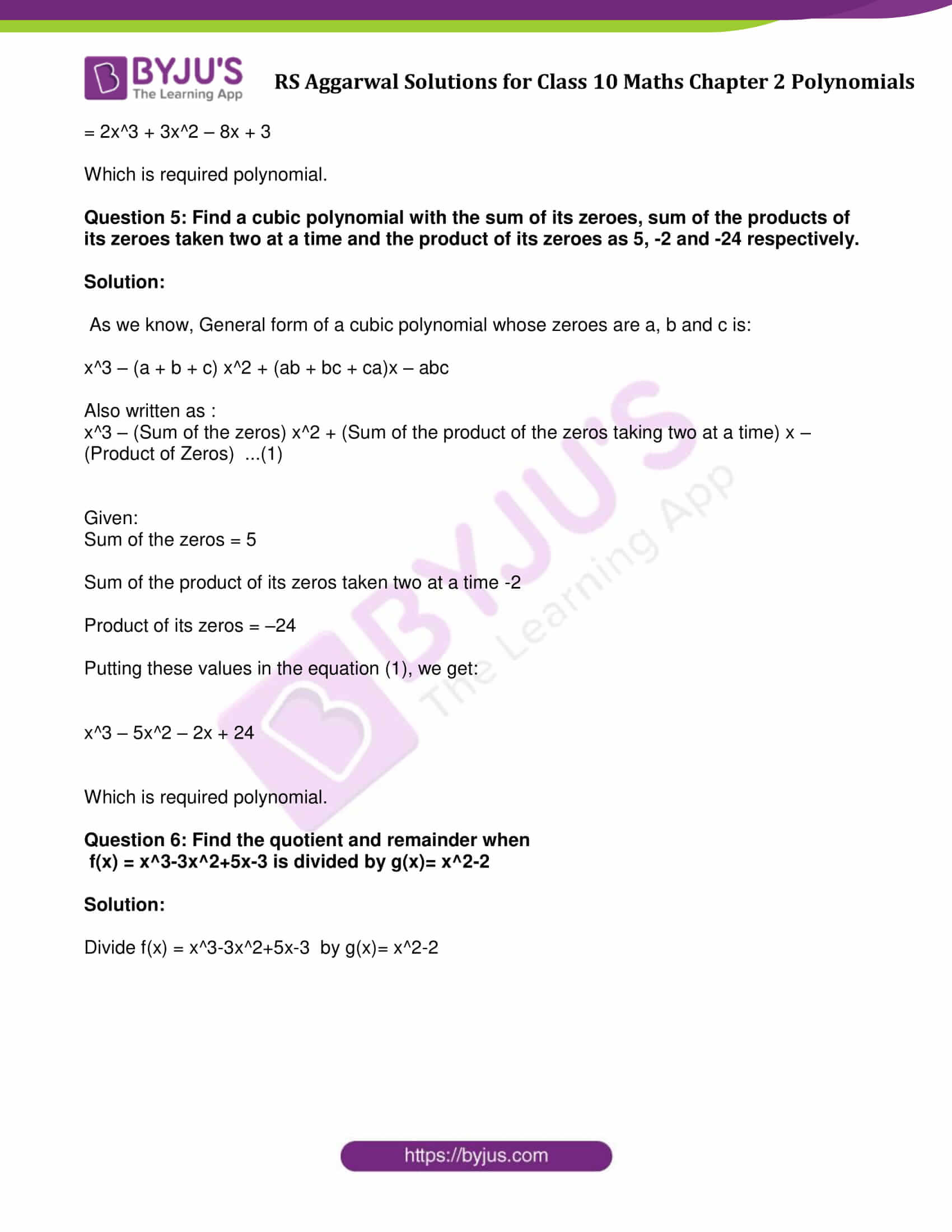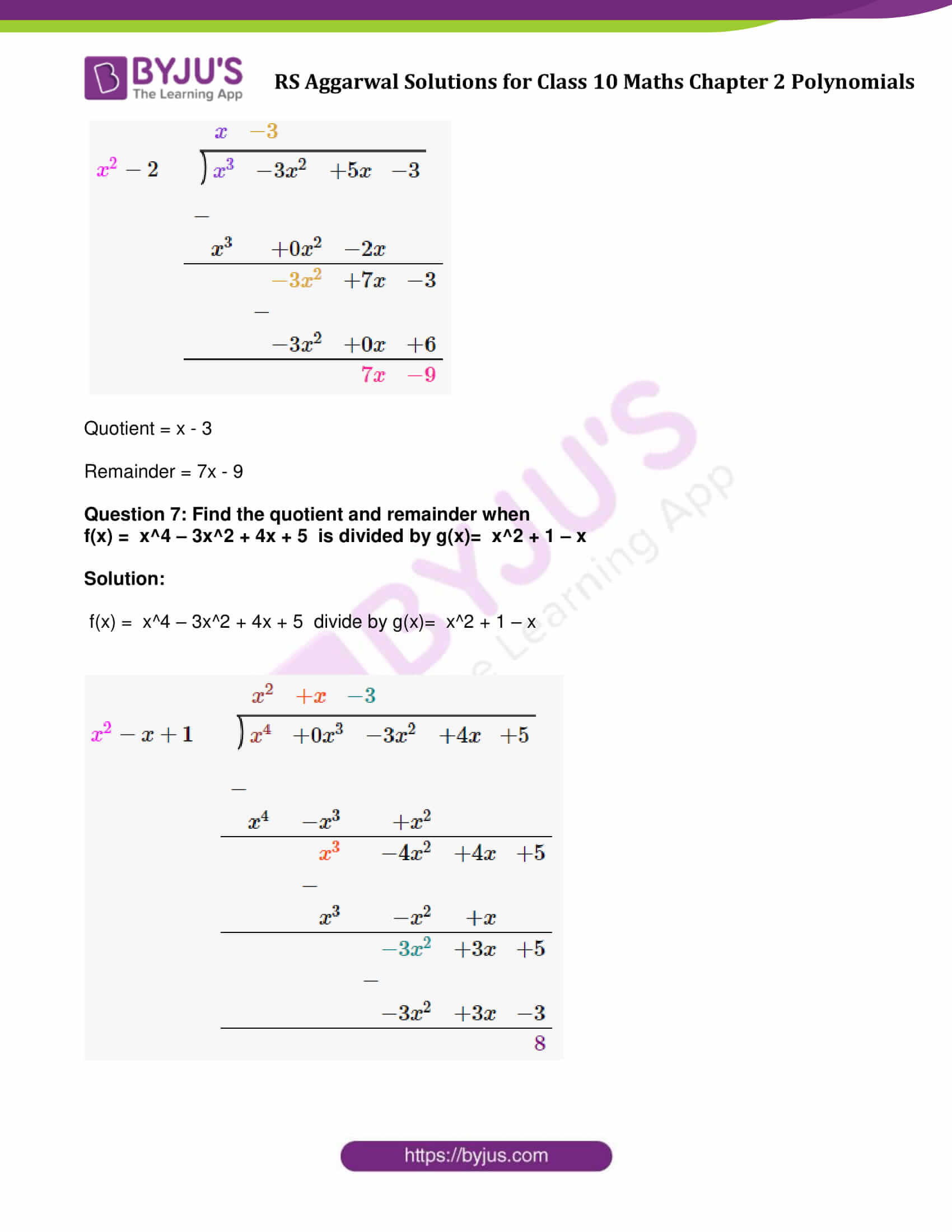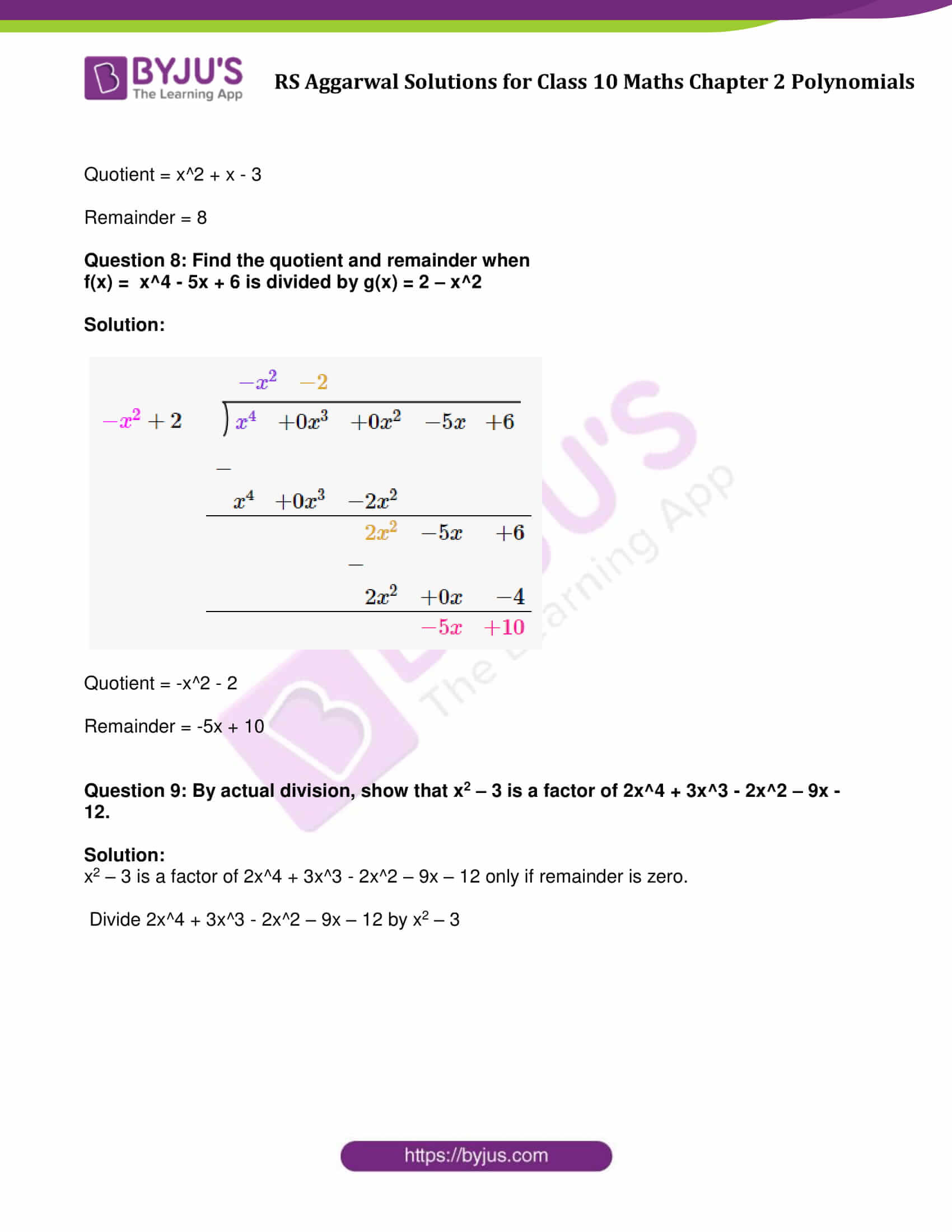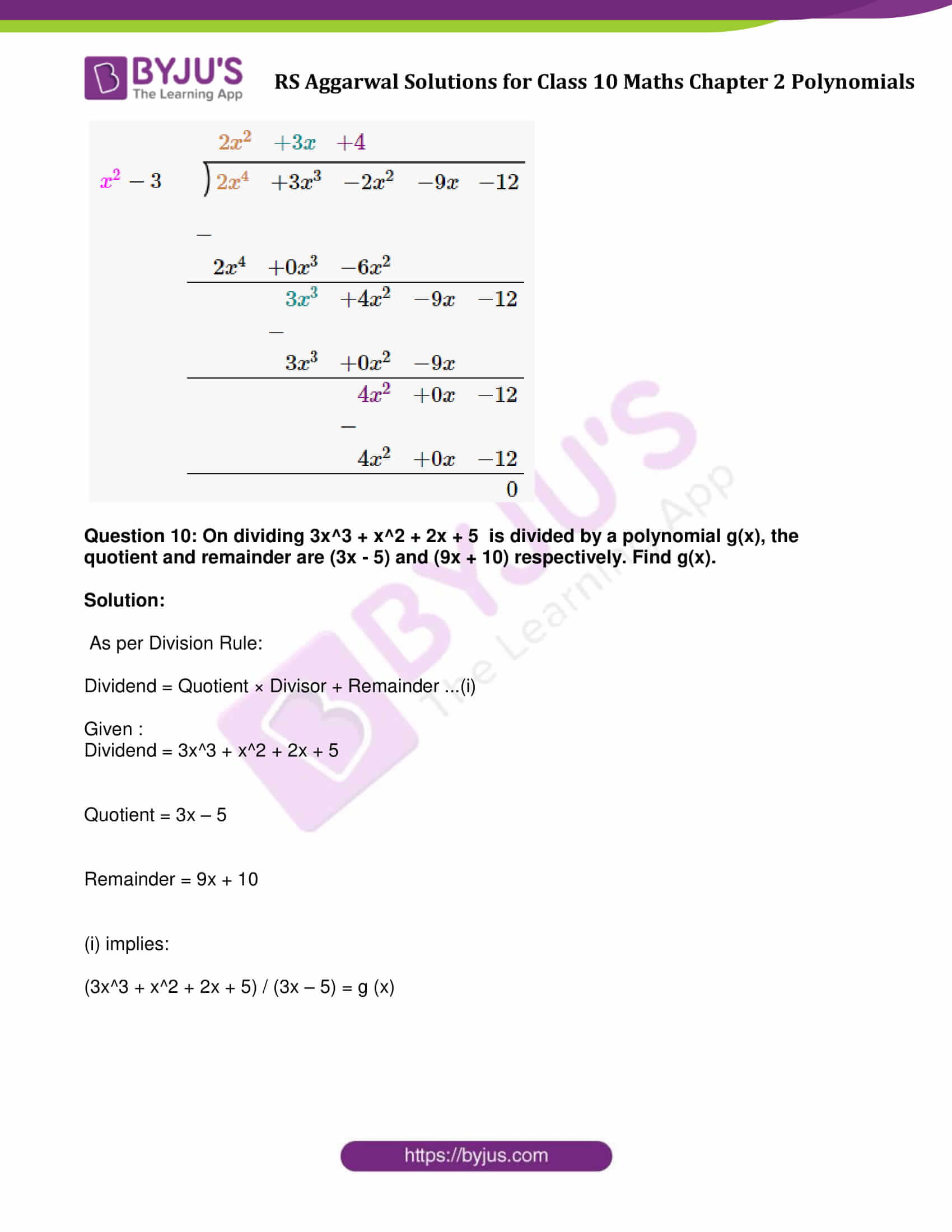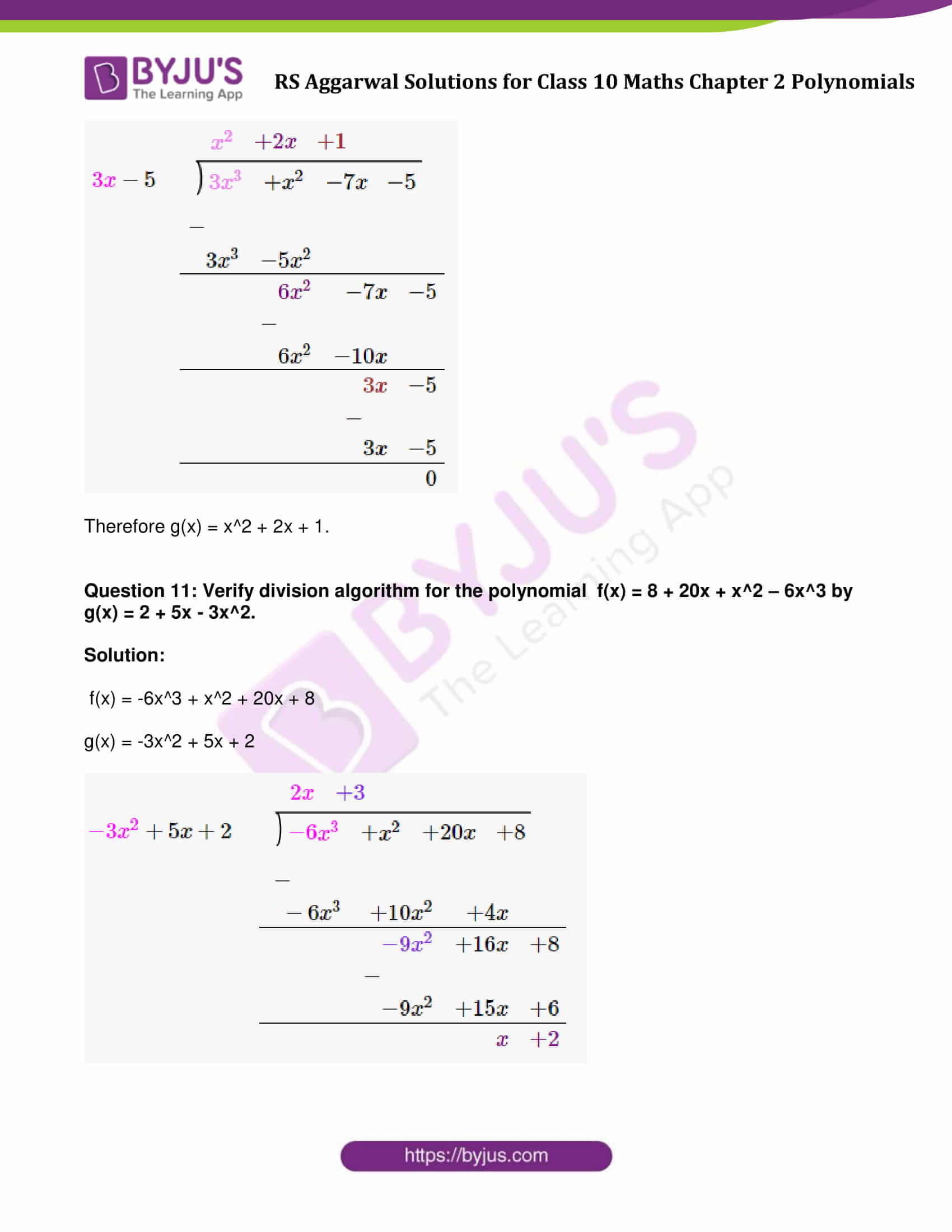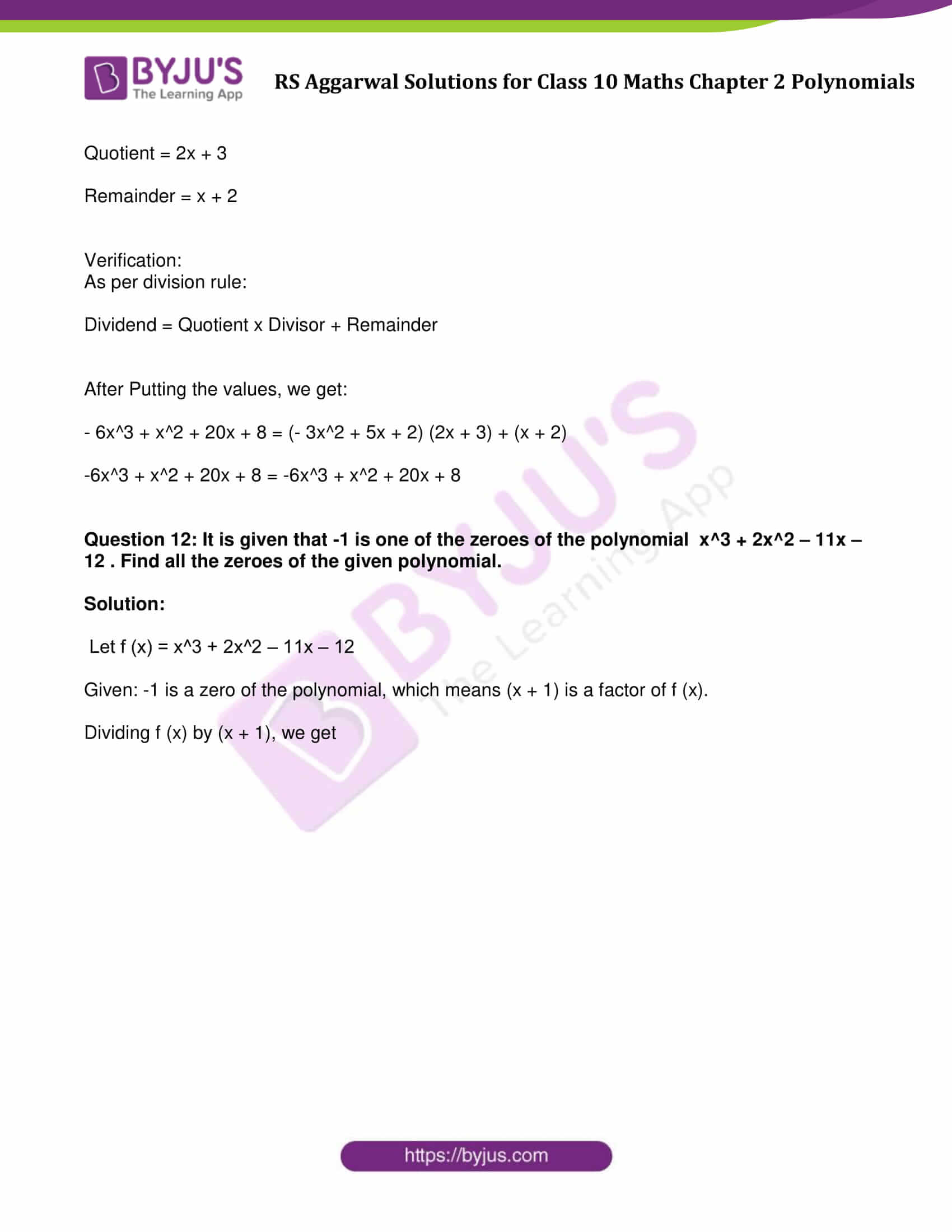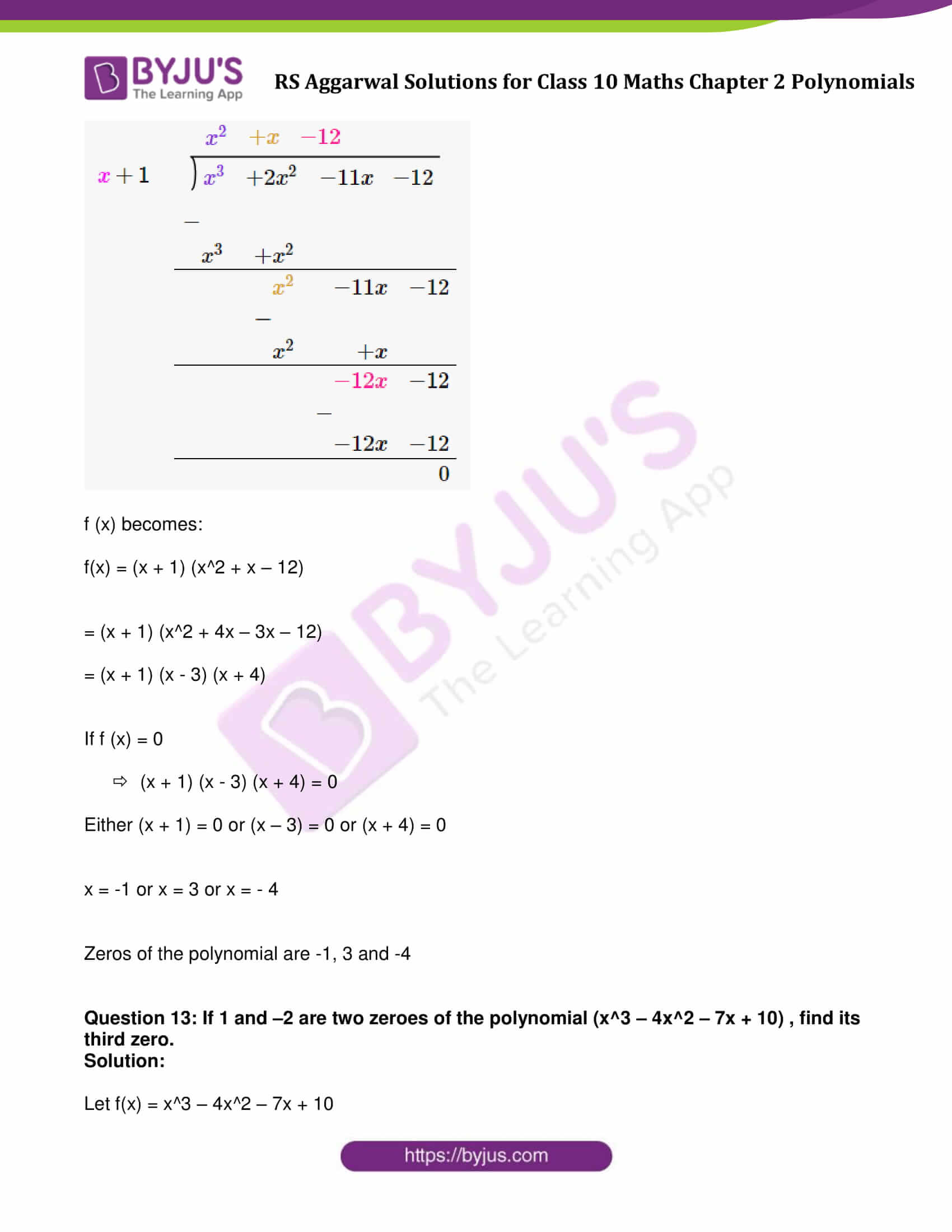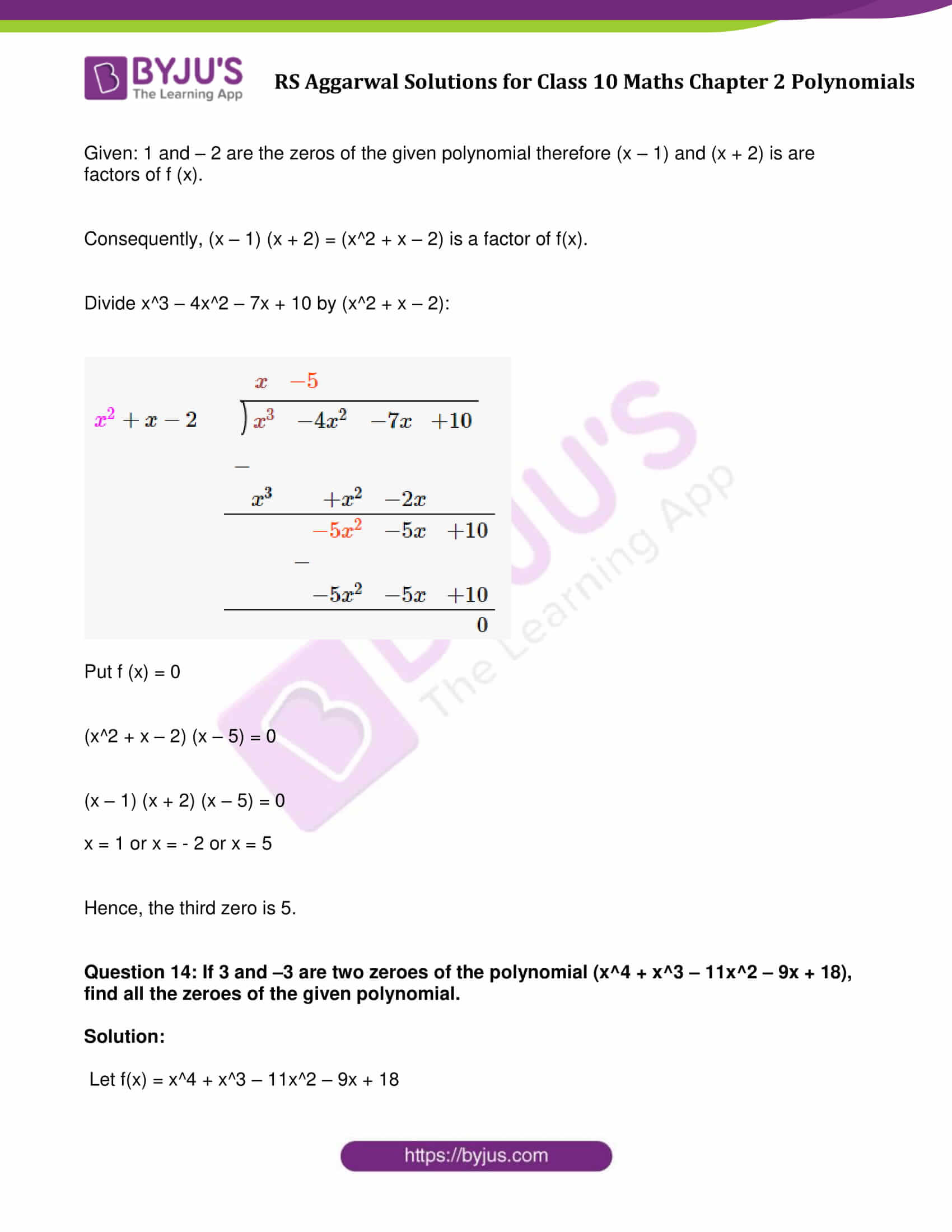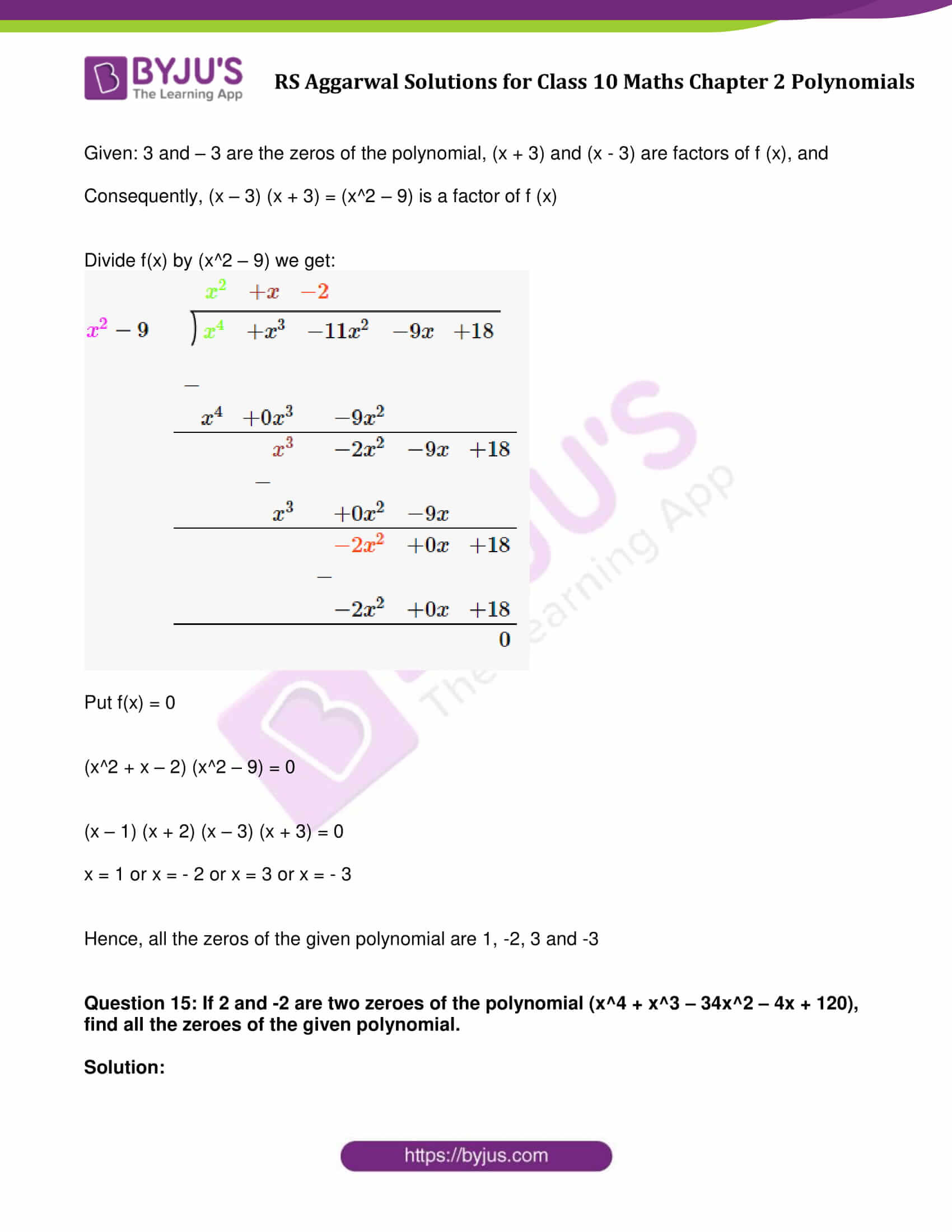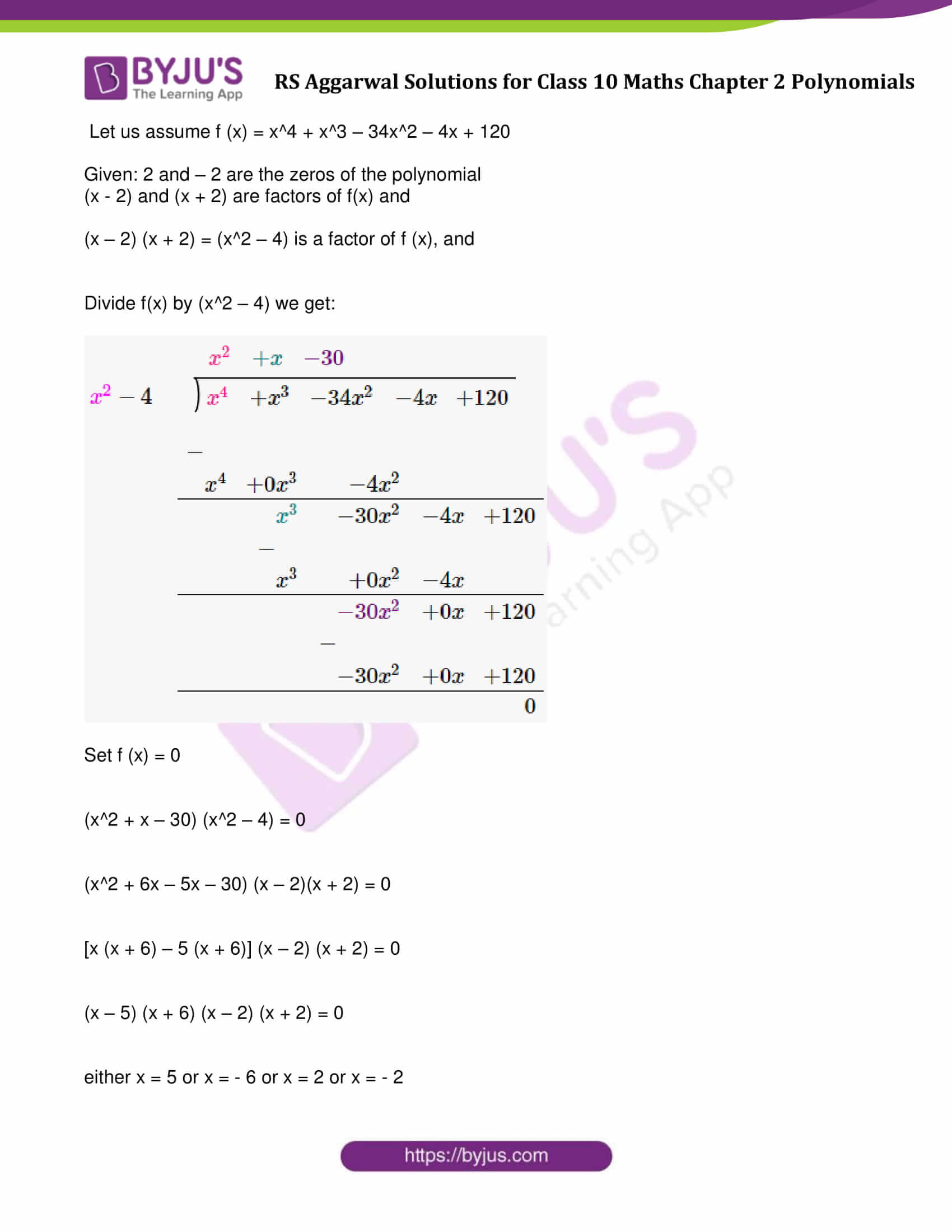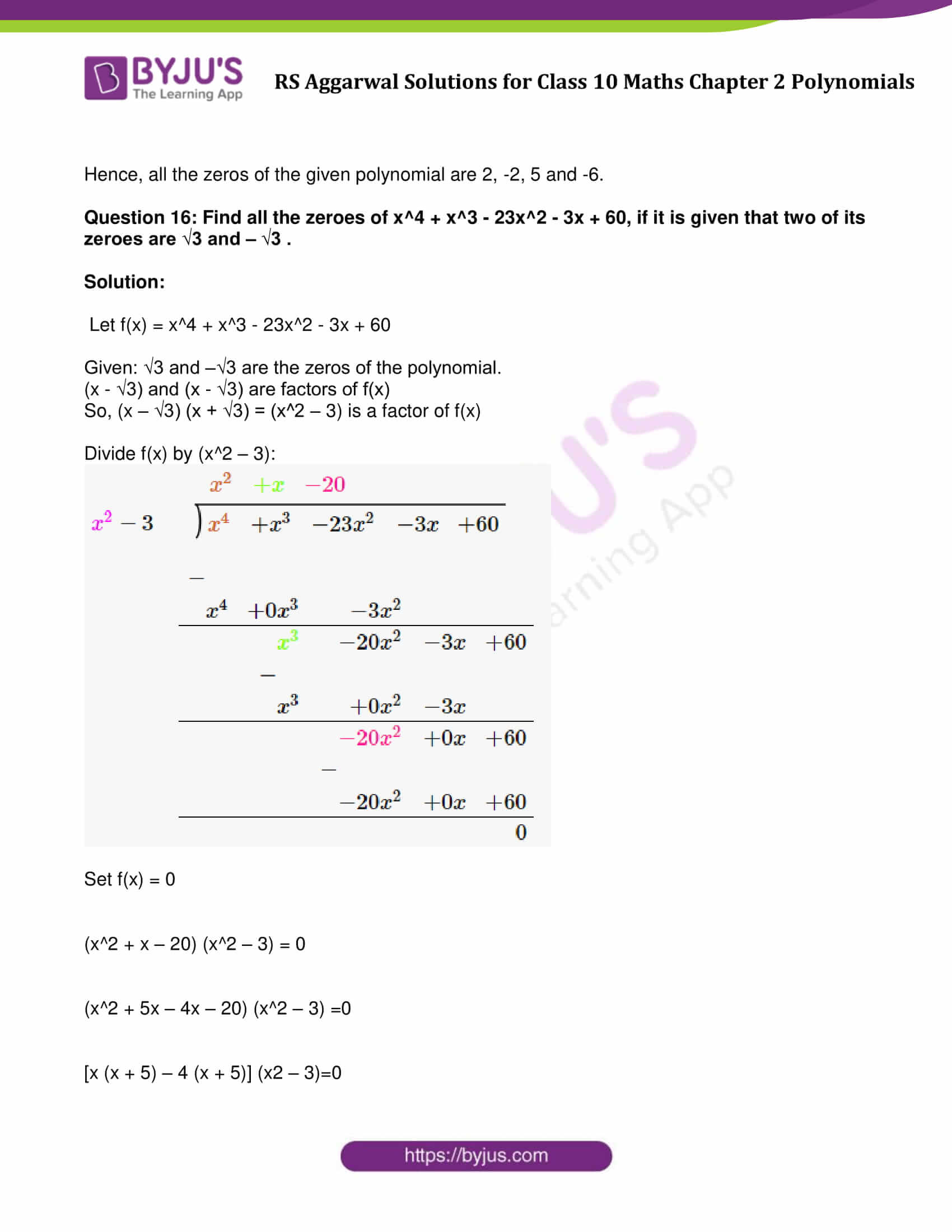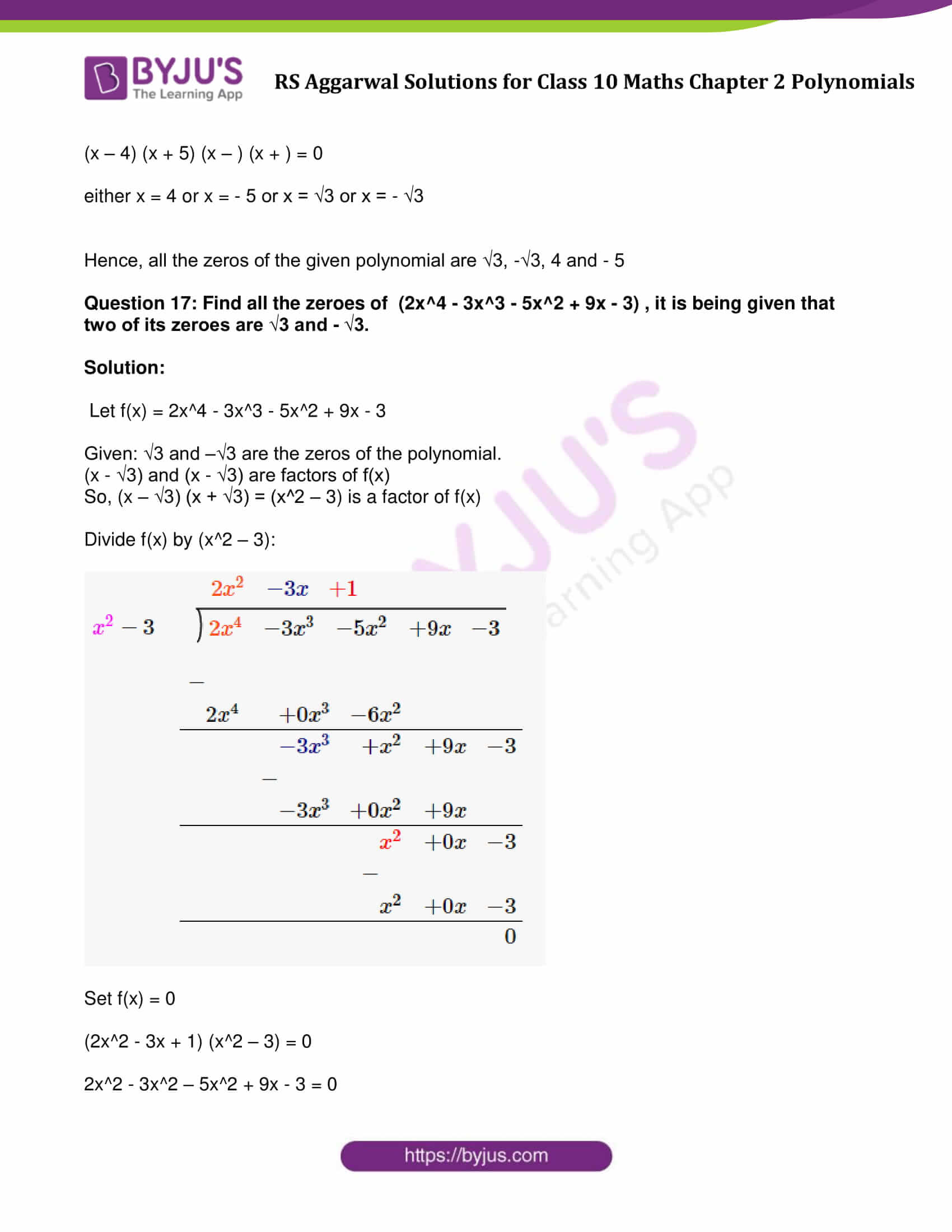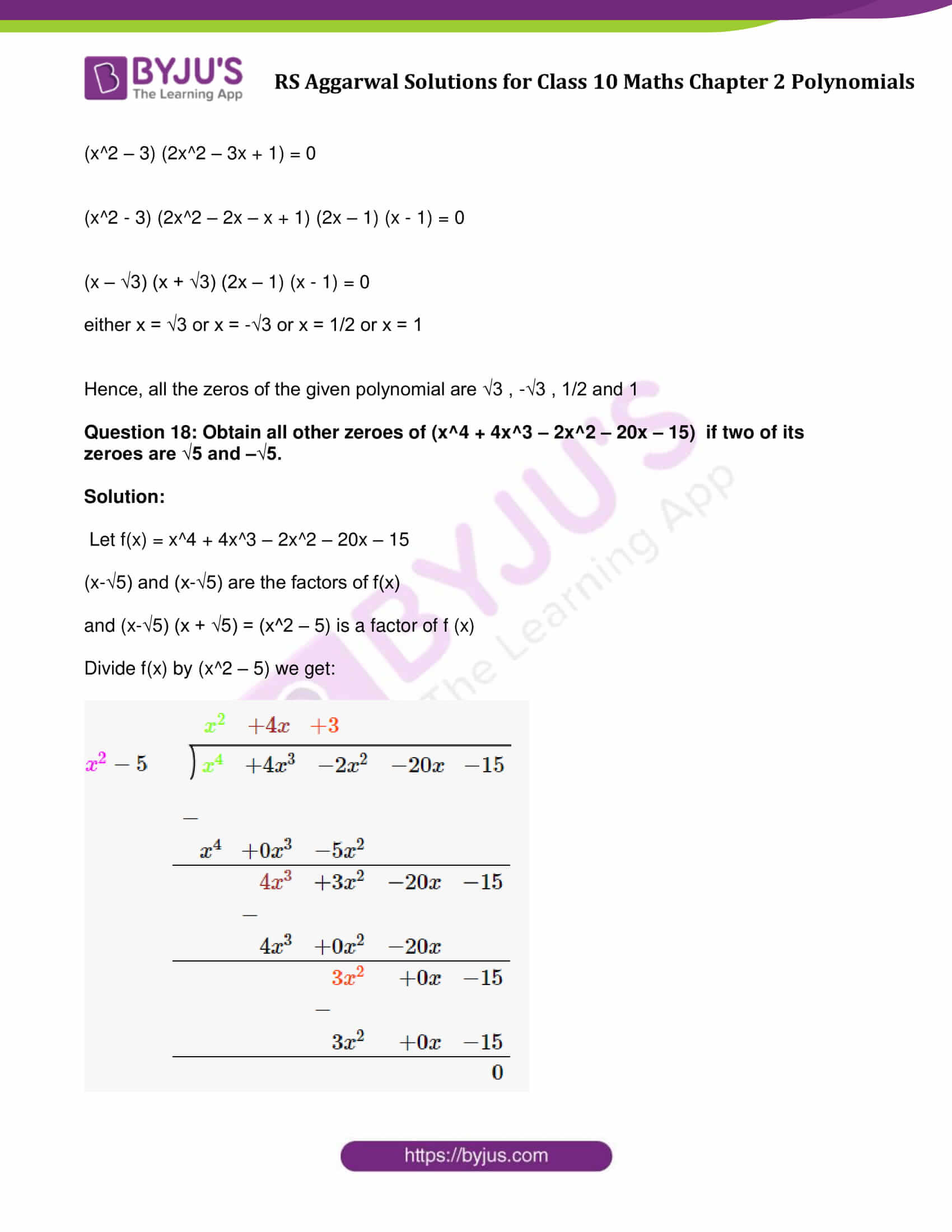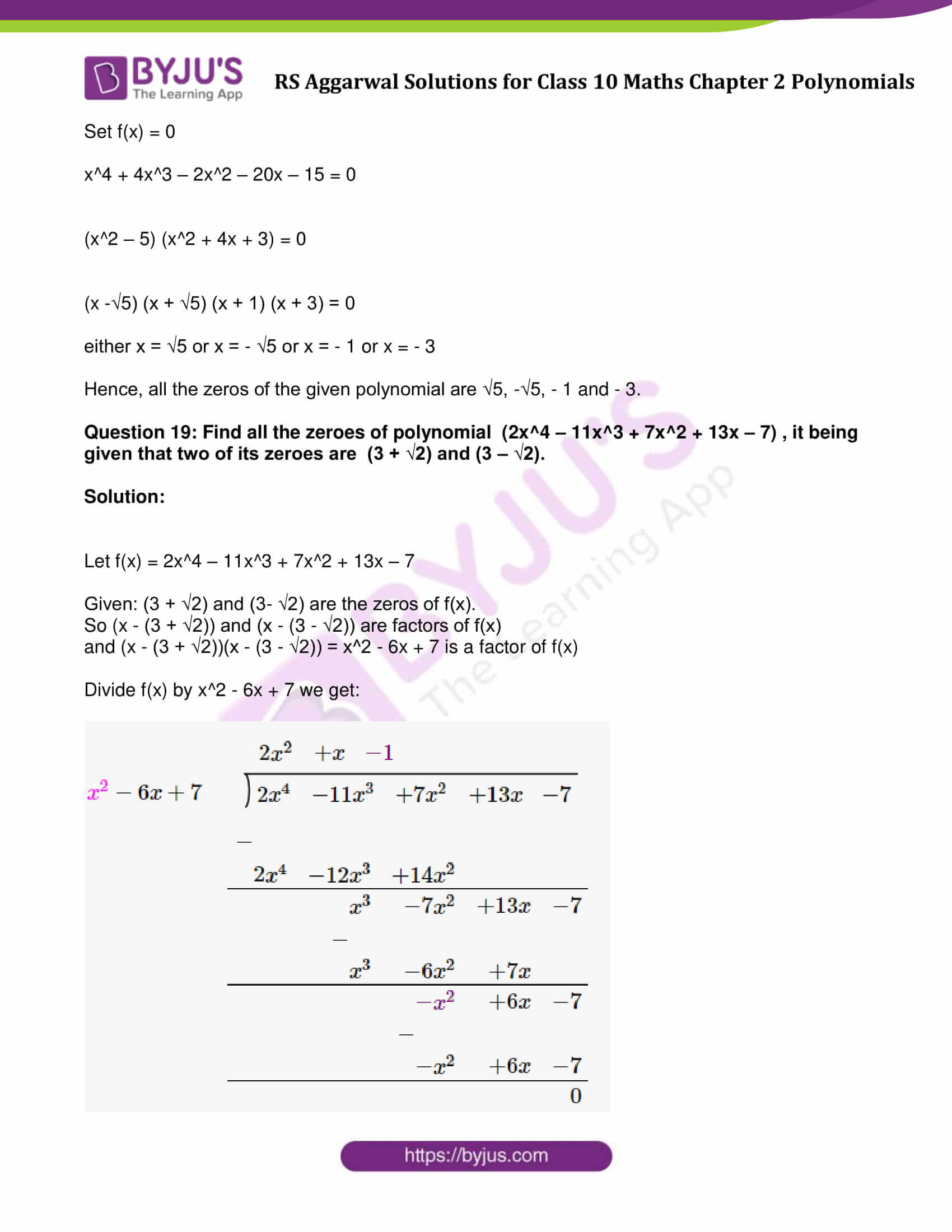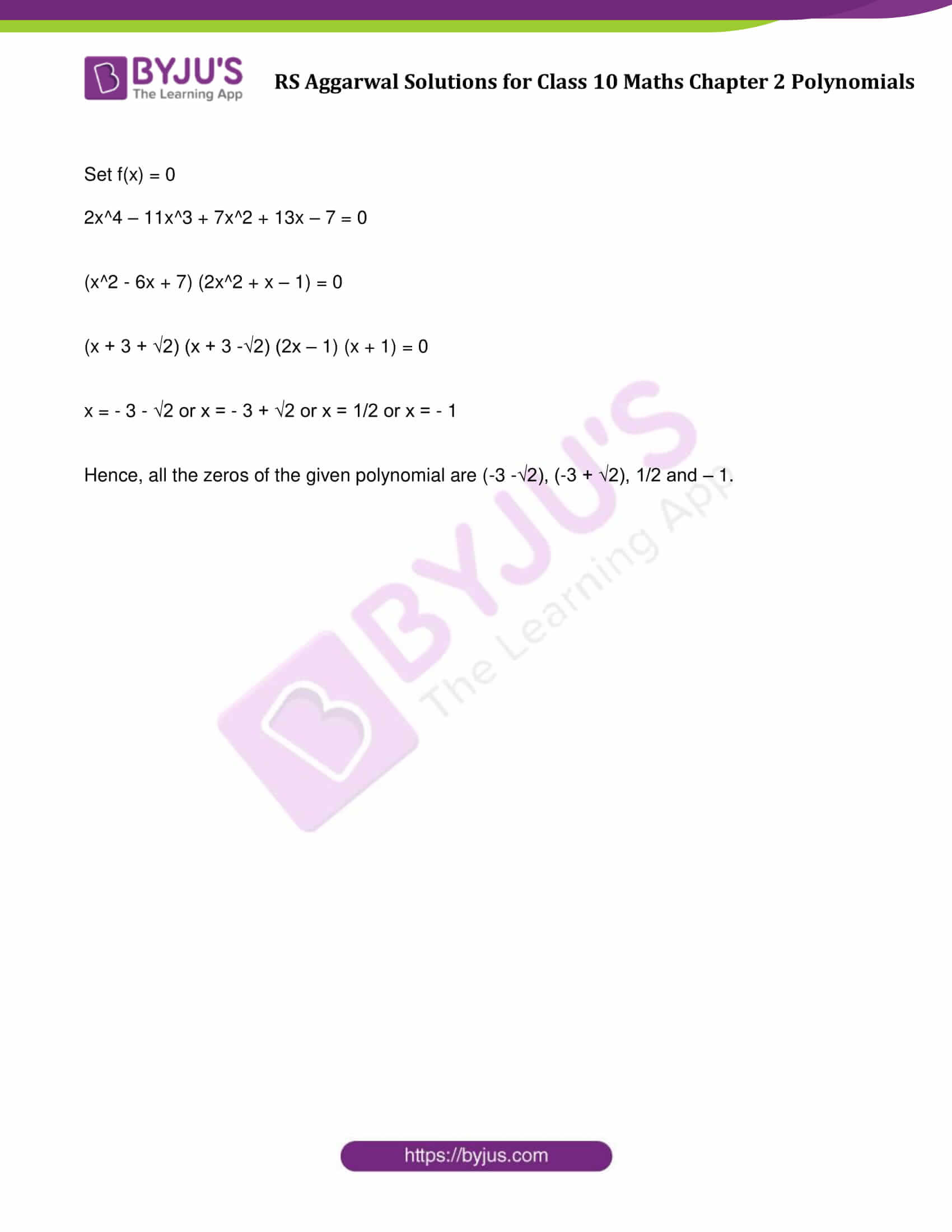### Access other exercise solutions of Class 10 Maths Chapter 2 Polynomials

Exercise 2A Solutions: 21 Questions (Short Answers)

Exercise 2C Solutions: 25 Questions (Short Answers)

## Exercise 2B Page No: 58

Question 1: Verify that 3, -2, 1 are the zeros of the cubic polynomial p(x) = (x^3 – 2x^2 – 5x + 6) and verify the relation between it zeros and coefficients.

Solution:

Let f(x) = x^3 – 2x^2 – 5x + 6

3, -2 and 1 are the zeros of the polynomial (given)

Therefore,

f(3) = (3)^3 – 2(3)^2 – 5(3) + 6

= 27 – 18 – 15 + 6

= 0

f(-2) = (-2)^3 – 2(-2)^2 – 5(-2) + 6

= -8 – 8 + 10 + 6

= 0

f(1) = (1)^3 – 2 (1)^2 – 5 (1) + 6

= 1 – 2 – 5 + 6

= 0

Verify relations:

General form of a cubic equation: ax^3 + bx^2 + cx + d.

Now,

Consider α = 3, β = – 2 and γ = 1

α + β + γ = 3 – 2 + 1 = 2 = -b/a

αβ + βγ + αγ = 3 (-2) + (-2) (1) + 1 (3) = -5 = c/a

And, αβγ = 3 (-2)(1) = -6 = -d/a

Question 2: Verify that 5, -2 and 1/3 are the zeroes of the cubic polynomial p(x) = (3x^3 – 10x^2 – 27x + 10) and verify the relation between its zeroes and coefficients.

Solution:

Let f(x) = 3x^3 – 10x^2 – 27x + 10

5, -2 and 1/3 are the zeros of the polynomial (given)

Therefore,

f(5) = 3(5)^3 – 10(5)^2 – 27(5) + 10

= 3 × 125 – 250 – 135 + 10

= 0

f(-2) = 3(-2)^3 – 10(-2)^2 – 27(-2) + 10

= – 24 – 40 + 54 + 10

= 0

f(1/3) = 3 (1/3)^3 – 10(1/3)^2 – 27(1/3) + 10

= 1/9 – 10/9 – 9 + 10

= 0

Verify relations:

General form of a cubic equation: ax^3 + bx^2 + cx + d.

Now,

Consider α = 5, β = – 2 and γ = 1/3

α + β + γ = 5 – 2 + 1/3 = 10/3 = -b/a

αβ + βγ + αγ = 5 (-2) + (-2) (1/3) + (1/3) (5) = -9 = c/a

And, αβγ = 5 (-2) (1/3)= -10/3 = -d/a

Question 3: Find a cubic polynomial whose zeroes are 2, -3 and 4.

Solution:

General form of a cubic polynomial whose zeroes are a, b and c is:

x^3 – (a + b + c) x^2 + (ab + bc + ca)x – abc …(1)

To Find: Cubic polynomial whose zeroes are 2, -3 and 4

Let us say, a = 2, b = – 3 and c = 4

Putting the values of a, b and c in the equation (1) we get:

= x^3 – (2 – 3 + 4) x^2 + (-6 – 12 + 8) x – (- 24)

= x^3 – 3x^2 – 10x + 24

Which is required polynomial.

Question 4: Find a cubic polynomial whose zeroes are 1/2, 1 and -3.

Solution:

General form of a cubic polynomial whose zeroes are a, b and c is:

x^3 – (a + b + c) x^2 + (ab + bc + ca)x – abc …(1)

To Find: Cubic polynomial whose zeroes are 1/2, 1 and -3

Let us say, a = 1/2, b = 1 and c = -3

Putting the values of a, b and c in the equation (1) we get:

= x^3 – (1/2 + 1 – 3) x^2 + (1/2 – 3 – 3/2)x – (- 3/2)

= x^3 – (-3/2) x^2 – 4x + 3/2

= 2x^3 + 3x^2 – 8x + 3

Which is required polynomial.

Question 5: Find a cubic polynomial with the sum of its zeroes, sum of the products of its zeroes taken two at a time and the product of its zeroes as 5, -2 and -24 respectively.

Solution:

As we know, General form of a cubic polynomial whose zeroes are a, b and c is:

x^3 – (a + b + c) x^2 + (ab + bc + ca)x – abc

Also written as :

x^3 – (Sum of the zeros) x^2 + (Sum of the product of the zeros taking two at a time) x – (Product of Zeros) …(1)

Given:

Sum of the zeros = 5

Sum of the product of its zeros taken two at a time -2

Product of its zeros = –24

Putting these values in the equation (1), we get:

x^3 – 5x^2 – 2x + 24

Which is required polynomial.

Question 6: Find the quotient and remainder when

f(x) = x^3-3x^2+5x-3 is divided by g(x)= x^2-2

Solution:

Divide f(x) = x^3-3x^2+5x-3 by g(x)= x^2-2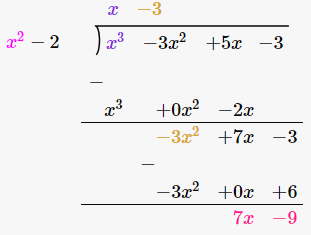Quotient = x – 3

Remainder = 7x – 9

Question 7: Find the quotient and remainder when

f(x) = x^4 – 3x^2 + 4x + 5 is divided by g(x)= x^2 + 1 – x

Solution:

f(x) = x^4 – 3x^2 + 4x + 5 divide by g(x)= x^2 + 1 – x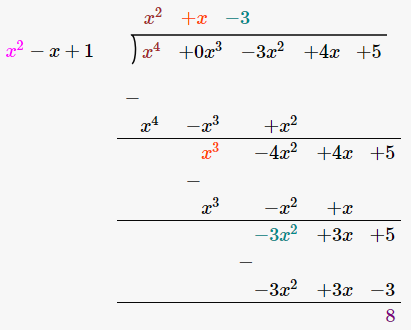Quotient = x^2 + x – 3

Remainder = 8

Question 8: Find the quotient and remainder when

f(x) = x^4 – 5x + 6 is divided by g(x) = 2 – x^2

Solution: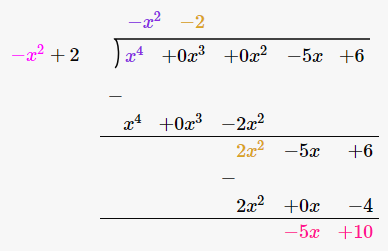Quotient = -x^2 – 2

Remainder = -5x + 10

Question 9: By actual division, show that x2 – 3 is a factor of 2x^4 + 3x^3 – 2x^2 – 9x – 12.

Solution:

x2 – 3 is a factor of 2x^4 + 3x^3 – 2x^2 – 9x – 12 only if remainder is zero.

Divide 2x^4 + 3x^3 – 2x^2 – 9x – 12 by x2 – 3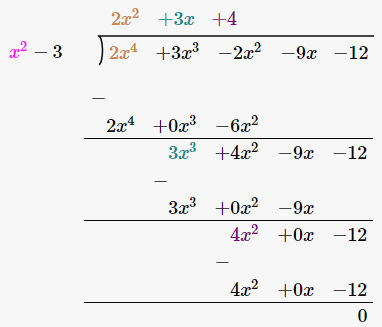Question 10: On dividing 3x^3 + x^2 + 2x + 5 is divided by a polynomial g(x), the quotient and remainder are (3x – 5) and (9x + 10) respectively. Find g(x).

Solution:

As per Division Rule:

Dividend = Quotient × Divisor + Remainder …(i)

Given :

Dividend = 3x^3 + x^2 + 2x + 5

Quotient = 3x – 5

Remainder = 9x + 10

(i) implies:

(3x^3 + x^2 + 2x + 5) / (3x – 5) = g (x)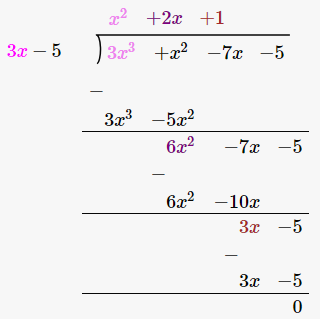Therefore g(x) = x^2 + 2x + 1.

Question 11: Verify division algorithm for the polynomial f(x) = 8 + 20x + x^2 – 6x^3 by g(x) = 2 + 5x – 3x^2.

Solution:

f(x) = -6x^3 + x^2 + 20x + 8

g(x) = -3x^2 + 5x + 2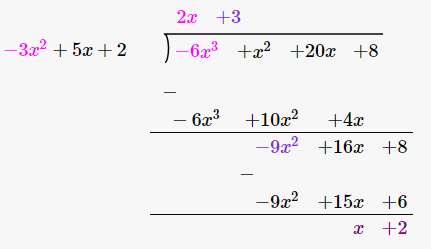Quotient = 2x + 3

Remainder = x + 2

Verification:

As per division rule:

Dividend = Quotient x Divisor + Remainder

After Putting the values, we get:

– 6x^3 + x^2 + 20x + 8 = (- 3x^2 + 5x + 2) (2x + 3) + (x + 2)

-6x^3 + x^2 + 20x + 8 = -6x^3 + x^2 + 20x + 8

Question 12: It is given that -1 is one of the zeroes of the polynomial x^3 + 2x^2 – 11x – 12 . Find all the zeroes of the given polynomial.

Solution:

Let f (x) = x^3 + 2x^2 ‒ 11x ‒ 12

Given: -1 is a zero of the polynomial, which means (x + 1) is a factor of f (x).

Dividing f (x) by (x + 1), we get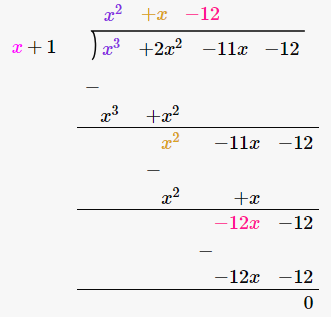f (x) becomes:

f(x) = (x + 1) (x^2 + x – 12)

= (x + 1) (x^2 + 4x – 3x – 12)

= (x + 1) (x – 3) (x + 4)

If f (x) = 0

• (x + 1) (x – 3) (x + 4) = 0

Either (x + 1) = 0 or (x – 3) = 0 or (x + 4) = 0

x = -1 or x = 3 or x = – 4

Zeros of the polynomial are -1, 3 and -4

Question 13: If 1 and –2 are two zeroes of the polynomial (x^3 – 4x^2 – 7x + 10) , find its third zero.

Solution:

Let f(x) = x^3 – 4x^2 – 7x + 10

Given: 1 and – 2 are the zeros of the given polynomial therefore (x – 1) and (x + 2) is are factors of f (x).

Consequently, (x – 1) (x + 2) = (x^2 + x – 2) is a factor of f(x).

Divide x^3 – 4x^2 – 7x + 10 by (x^2 + x – 2):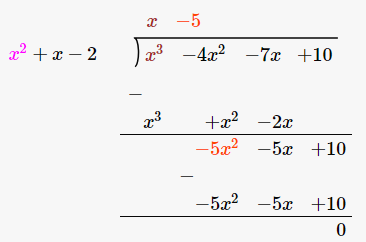Put f (x) = 0

(x^2 + x – 2) (x – 5) = 0

(x – 1) (x + 2) (x – 5) = 0

x = 1 or x = – 2 or x = 5

Hence, the third zero is 5.

Question 14: If 3 and –3 are two zeroes of the polynomial (x^4 + x^3 – 11x^2 – 9x + 18), find all the zeroes of the given polynomial.

Solution:

Let f(x) = x^4 + x^3 – 11x^2 – 9x + 18

Given: 3 and – 3 are the zeros of the polynomial, (x + 3) and (x – 3) are factors of f (x), and

Consequently, (x – 3) (x + 3) = (x^2 – 9) is a factor of f (x)

Divide f(x) by (x^2 – 9) we get: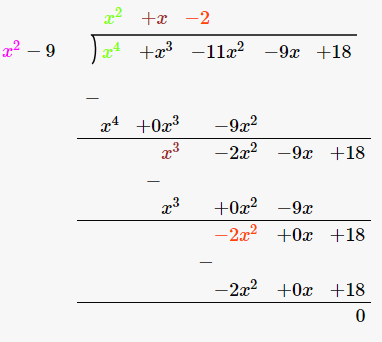Put f(x) = 0

(x^2 + x – 2) (x^2 – 9) = 0

(x – 1) (x + 2) (x – 3) (x + 3) = 0

x = 1 or x = – 2 or x = 3 or x = – 3

Hence, all the zeros of the given polynomial are 1, -2, 3 and -3

Question 15: If 2 and -2 are two zeroes of the polynomial (x^4 + x^3 – 34x^2 – 4x + 120), find all the zeroes of the given polynomial.

Solution:

Let us assume f (x) = x^4 + x^3 – 34x^2 – 4x + 120

Given: 2 and – 2 are the zeros of the polynomial

(x – 2) and (x + 2) are factors of f(x) and

(x – 2) (x + 2) = (x^2 – 4) is a factor of f (x), and

Divide f(x) by (x^2 – 4) we get: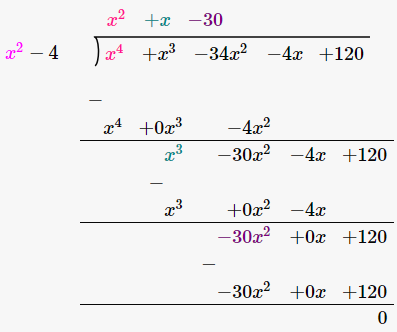Set f (x) = 0

(x^2 + x – 30) (x^2 – 4) = 0

(x^2 + 6x – 5x – 30) (x – 2)(x + 2) = 0

[x (x + 6) – 5 (x + 6)] (x – 2) (x + 2) = 0

(x – 5) (x + 6) (x – 2) (x + 2) = 0

either x = 5 or x = – 6 or x = 2 or x = – 2

Hence, all the zeros of the given polynomial are 2, -2, 5 and -6.

Question 16: Find all the zeroes of x^4 + x^3 – 23x^2 – 3x + 60, if it is given that two of its zeroes are √3 and – √3 .

Solution:

Let f(x) = x^4 + x^3 – 23x^2 – 3x + 60

Given: √3 and –√3 are the zeros of the polynomial.

(x – √3) and (x – √3) are factors of f(x)

So, (x – √3) (x + √3) = (x^2 – 3) is a factor of f(x)

Divide f(x) by (x^2 – 3):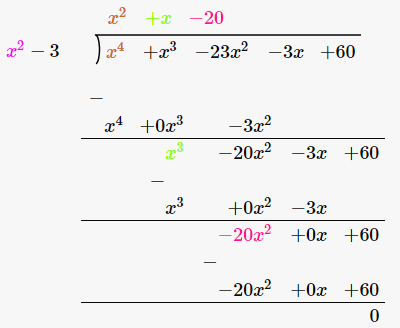Set f(x) = 0

(x^2 + x – 20) (x^2 – 3) = 0

(x^2 + 5x – 4x – 20) (x^2 – 3) =0

[x (x + 5) – 4 (x + 5)] (x2 – 3)=0

(x – 4) (x + 5) (x – ) (x + ) = 0

either x = 4 or x = – 5 or x = √3 or x = – √3

Hence, all the zeros of the given polynomial are √3, -√3, 4 and – 5

Question 17: Find all the zeroes of (2x^4 – 3x^3 – 5x^2 + 9x – 3) , it is being given that two of its zeroes are √3 and – √3.

Solution:

Let f(x) = 2x^4 – 3x^3 – 5x^2 + 9x – 3

Given: √3 and –√3 are the zeros of the polynomial.

(x – √3) and (x – √3) are factors of f(x)

So, (x – √3) (x + √3) = (x^2 – 3) is a factor of f(x)

Divide f(x) by (x^2 – 3):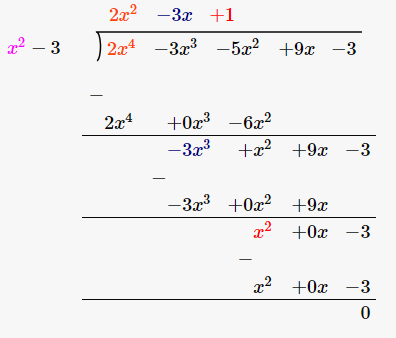Set f(x) = 0

(2x^2 – 3x + 1) (x^2 – 3) = 0

2x^2 – 3x^2 – 5x^2 + 9x – 3 = 0

(x^2 – 3) (2x^2 – 3x + 1) = 0

(x^2 – 3) (2x^2 – 2x – x + 1) (2x – 1) (x – 1) = 0

(x – √3) (x + √3) (2x – 1) (x – 1) = 0

either x = √3 or x = -√3 or x = 1/2 or x = 1

Hence, all the zeros of the given polynomial are √3 , -√3 , 1/2 and 1

Question 18: Obtain all other zeroes of (x^4 + 4x^3 – 2x^2 – 20x – 15) if two of its zeroes are √5 and –√5.

Solution:

Let f(x) = x^4 + 4x^3 – 2x^2 – 20x – 15

(x-√5) and (x-√5) are the factors of f(x)

and (x-√5) (x + √5) = (x^2 – 5) is a factor of f (x)

Divide f(x) by (x^2 – 5) we get: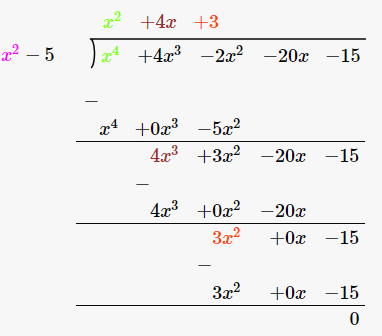Set f(x) = 0

x^4 + 4x^3 – 2x^2 – 20x – 15 = 0

(x^2 – 5) (x^2 + 4x + 3) = 0

(x -√5) (x + √5) (x + 1) (x + 3) = 0

either x = √5 or x = – √5 or x = – 1 or x = – 3

Hence, all the zeros of the given polynomial are √5, -√5, – 1 and – 3.

Question 19: Find all the zeroes of polynomial (2x^4 – 11x^3 + 7x^2 + 13x – 7) , it being given that two of its zeroes are (3 + √2) and (3 – √2).

Solution:

Let f(x) = 2x^4 – 11x^3 + 7x^2 + 13x – 7

Given: (3 + √2) and (3- √2) are the zeros of f(x).

So (x – (3 + √2)) and (x – (3 – √2)) are factors of f(x)

and (x – (3 + √2))(x – (3 – √2)) = x^2 – 6x + 7 is a factor of f(x)

Divide f(x) by x^2 – 6x + 7 we get:Set f(x) = 0

2x^4 – 11x^3 + 7x^2 + 13x – 7 = 0

(x^2 – 6x + 7) (2x^2 + x – 1) = 0

(x + 3 + √2) (x + 3 -√2) (2x – 1) (x + 1) = 0

x = – 3 – √2 or x = – 3 + √2 or x = 1/2 or x = – 1

Hence, all the zeros of the given polynomial are (-3 -√2), (-3 + √2), 1/2 and – 1.

## RS Aggarwal Solutions for Class 10 Maths Chapter 2 Polynomials Exercise 2B

Class 10 Maths Chapter 2 Polynomials Exercise 2B is based on polynomials and relation between the zeros and coefficients of a quadratic Polynomial.